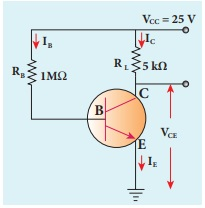Home | | Physics 12th Std | Operating Point

# Operating Point

The operating point is a point where the transistor can be operated efficiently.

Operating Point

The operating point is a point where the transistor can be operated efficiently. A line that is drawn with the values VCC (when IC = 0) and IC (when VCE = 0) is called the dc load line. The dc load line superimposed on the output characteristics of a transistor is used to learn the operating point of the transistor as shown in Figure 9.35.Points P, Q, R in Figure 9.35 are called Q points or quiescent points which determine the operating point or the working point of a transistor. If the operating point is chosen at the middle of the dc load line (point Q), the transistor can effectively work as an amplifier. The operating point determines the maximum signal that can be obtained without being distorted.

For a transistor to work as a open switch, the Q point can be chosen at the cut-off region and to work as a closed switch, the Q point can be chosen in the saturation region.

EXAMPLE: 9.8

The current gain of a common emitter transistor circuit shown in figure is 120. Draw the dc load line and mark the Q point on it. (VBE to be ignored).Solution

Î˛ = 120Tags : Bipolar Junction Transistor [BJT] , 12th Physics : UNIT 10a : Semiconductor Electronics
Study Material, Lecturing Notes, Assignment, Reference, Wiki description explanation, brief detail
12th Physics : UNIT 10a : Semiconductor Electronics : Operating Point | Bipolar Junction Transistor [BJT]